# How to generate Gaussian samples

## Part 1: Inverse transform sampling

• To see the code I wrote for this project, you can check out its Github repo
• For other parts of the project: part 1, part 2, part 3

# Background

Gaussian sampling — that is, generating samples from a Gaussian distribution — plays an important role in many cutting-edge fields of data science, such as Gaussian process, variational autoencoder, or generative adversarial network. As a result, you often see functions like tf.random.normal in their tutorials.

But, deep down, how does a computer know how to generate Gaussian samples? This series of blog posts will show 3 different ways that we can program our computer (via Python) to do so. You will also see how R and Python generate Gaussian samples using modified versions of some of these methods.

# Starting point: the uniform number generator

Of course, we can’t generate Gaussian samples from thin air. Instead, we start with a random number generator that exists in almost all programming languages: the uniform random number generator. It generates a random number that could take any value between 0 and 1. For Python, the numpy.random module uses the Mersenne twister to generate a uniformly-distributed float that is in the interval [0.0, 1.0).

Since Gaussians are better visualized in 2 dimensions — we are all familiar with the Gaussian “blob” in the xy-plane — I will demonstrate the 3 sampling methods in 2-D, especially since one of the methods do in fact generate Gaussians in two dimensions at the same time.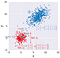Step 1: Generate standard Gaussian samples in 2-D. Step 2: Transform standard Gaussian samples to have given means, variances, and covariance between x and y

As a result, this series is broken down into 3 parts (see accompanying image):

1. The first two part — part 1 and part 2 — describes two common methods to transform the uniform samples (in gray) into points in which their x- and y-coordinates are independent standard Gaussians: both coordinates have mean of 0, variance of 1, and their covariance is 0 (in red). The first method, the inverse transform sampling, is described below.
2. The last part — part 3 — reveals a much simpler alternative to the the two methods above in generating standard Gaussian samples. It will also show how to transform these 2-D standard Gaussian samples (in red) to have any given mean or variance in x and y, as well as any given covariance between the x and y coordinates (in blue).

# Method 1: Inverse transform sampling

This is the most basic, and arguably most common, way to convert a uniform random sample into a random sample of any distribution, including Gaussian. This method works by applying the inverse function of the Gaussian CDF (cumulative distribution function) to transform a uniform sample to a Gaussian sample.

To make sure that the Gaussian samples for the x- and y-coordinates are independent, we can use two different uniform samples, one for x (U₁), and one for y (U₂). These two uniform samples can be generated using two different random number generators (two different `RandomState` initialized by different seeds, for example) so that they are independent in the first place.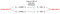Generate 2-D Gaussian samples from uniform samples

## How does this work?

This method works by exploiting a mind-blowing principle:

For any distribution, the cumulative probability is always uniformly distributed.

The arithmetic proof of this principle is straight-forward but rather boring, and you can view it from this lecture. Instead, I will show the geometric interpretation of this principle. But first, let’s clarify what cumulative probability is:

• The cumulative probability at some value x is the probability that the random variable X has a value that is lower than x: P(X < x). This can be obtained by integrating the probability density function (PDF)* of X from negative infinity to x. Geometrically, this is the area under the PDF to the left of x, which I will call the “left-side area” from here on (see green area in the graph below).
• As a result, for any value of x, we can always calculate its cumulative probability / left-side area. The function that converts the value x into its cumulative probability is appropriately called the cumulative distribution function (CDF)*. In the below graph for Gaussian distribution, the left-side area at x=0 is of course 0.5; alternatively, we can infer this area by looking at the CDF at x=0, which also comes out to be 0.5 (see the red points).

* Technically, PDF and CDF are functions that transform one variable to another, while density and cumulative probability are outputs of these functions respectively. However, the word PDF is often used interchangeably with density, and CDF with cumulative probability.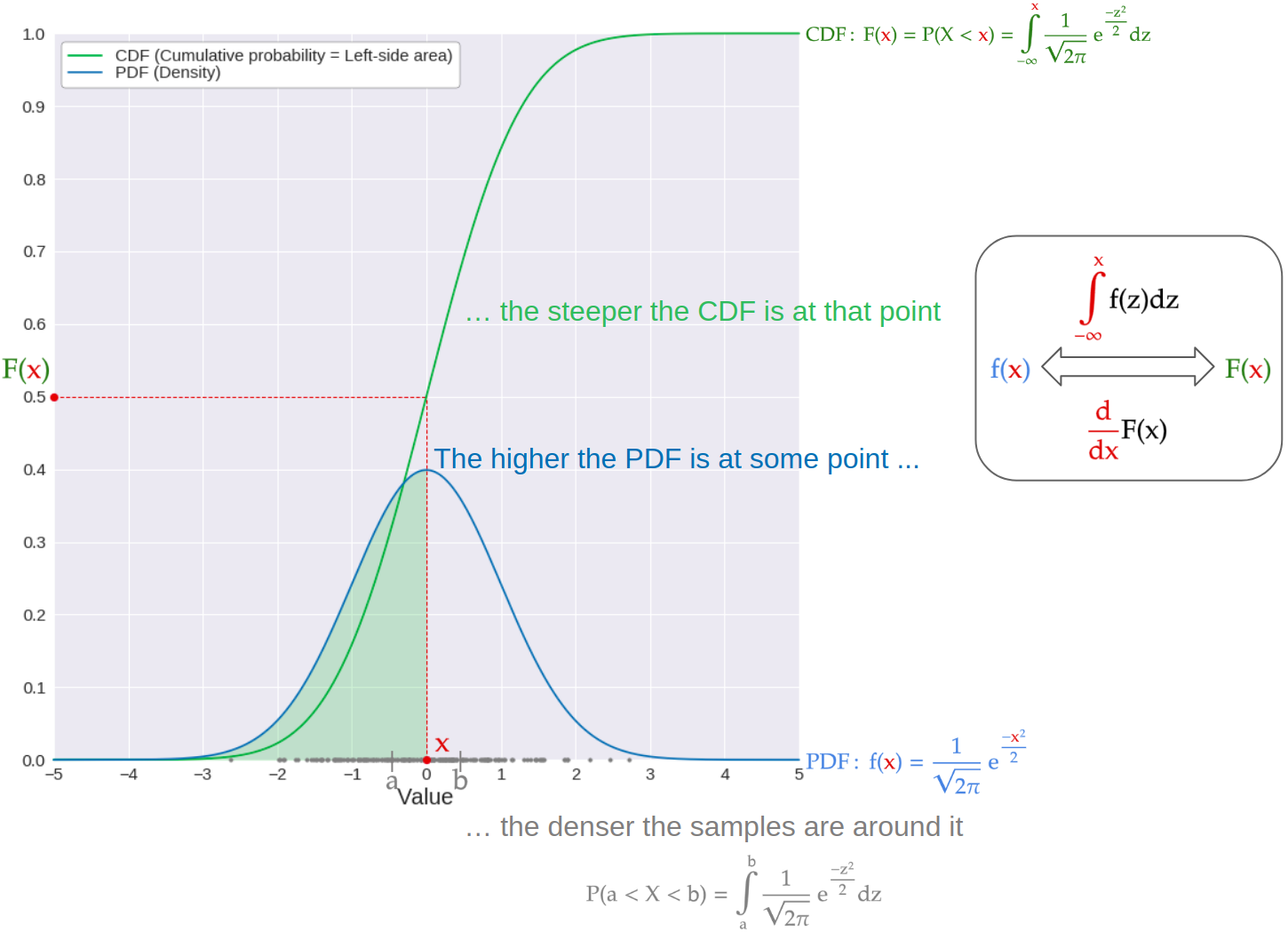Relationship between Gaussian PDF, CDF, and generated samples

Knowing what the PDF (density) and the CDF (cumulative probability / left-side area) are, we observe some interesting relationships between them. More specifically, the higher the density at some point

• the denser the Gaussian samples are around that point. This is because we can integrate the PDF over a small interval (a, b) around that point to get the probability that a Gaussian sample will lie within this interval. As a result, if the density in this interval is high, the probability that a Gaussian sample falls within this interval is also high.
• the steeper the CDF is at that point. As mentioned earlier, the CDF can be found by integrating the PDF with the appropriate bounds. As a result, the PDF itself is the derivative of the CDF, and represents the slope of the CDF at a given point.

These observations allow us to visually interpret the following simulation:

1. Generate 1000 samples from a standard Gaussian — also called N(0, 1) from its mean of 0 and standard deviation of 1— and plot histogram of the sampled Gaussian samples.
2. Use the Gaussian CDF — gray line in lower left graph — to calculate the cumulative probability of each sampled Gaussian. This is also the area under the PDF to the right of each sample (see upper right graph). We then plot the histogram of these areas.

As seen from the above animation of generating these 1000 Gaussian samples:

• The generated samples are indeed standard Gaussians, as they are very dense near x=0, and become less dense the further we move away from zero.
• However, when we trace each sampled value down to the CDF of N(0, 1), then rightward to get the left-side area of that value, we see that the dense samples near zero happen to hit the CDF at its steepest part. This part, in turn, collects many of these samples and distributes them evenly rightward.
• As a result, even though the samples are themselves Gaussian, their corresponding left-side areas are uniformly distributed! In other words, a left-side area of, say, 0.46, has an equal chance to occur as an area of 0.02, or an area of 0.99.

In short, this counter-intuitive principle allows us to transform a sample from any distribution into a uniform sample, using the CDF of that distribution. However, it is much more useful in the reverse direction: if we have a uniform sample, we can transform it back to a sample from any distribution we’d like. This is done, of course, by applying the inverse function of the distribution’s CDF to the uniform sample — hence the name “inverse transform sampling”.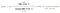Convert Gaussian sample to left-side area, and vice versa

Now that we are know this powerful and versatile sampling method, the remaining step is to find the inverse CDF of N(0, 1). However, this turns out to be much harder than expected!

# The CDF of standard Gaussian

For some distributions, such as the exponential, the inverse CDF is easy to find. In contrast, for standard Gaussian, this is far from the case. As a result, before finding the inverse CDF of N(0, 1), let’s first find the CDF of the distribution.

As mentioned earlier, to find the CDF at any point x, we just integrate the PDF from negative infinity to that point. For the standard Gaussian distribution, we can break this integral down into two smaller integrals: one from negative infinity to zero, and the other from zero to x. Hence, the first integral conveniently becomes 1/2 — see orange area under the PDF — since the standard Gaussian PDF is symmetric around zero.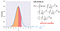Left: PDF of standard gaussian

The second integral — red area under the PDF — is where the headache starts, since despite its deceptively simple form, there is no way to evaluate it! In other words, there’s no additional step that we can take from this point on to evaluate or simplify the CDF of N(0, 1).

However, if one looks up the CDF of the standard Gaussian distribution, the most common formula for the CDF involves the strange error function (erf). Don’t worry: the error function itself is nothing but a modified version of our solution-less integral above, obtained by a small change of variable within the integration (see below).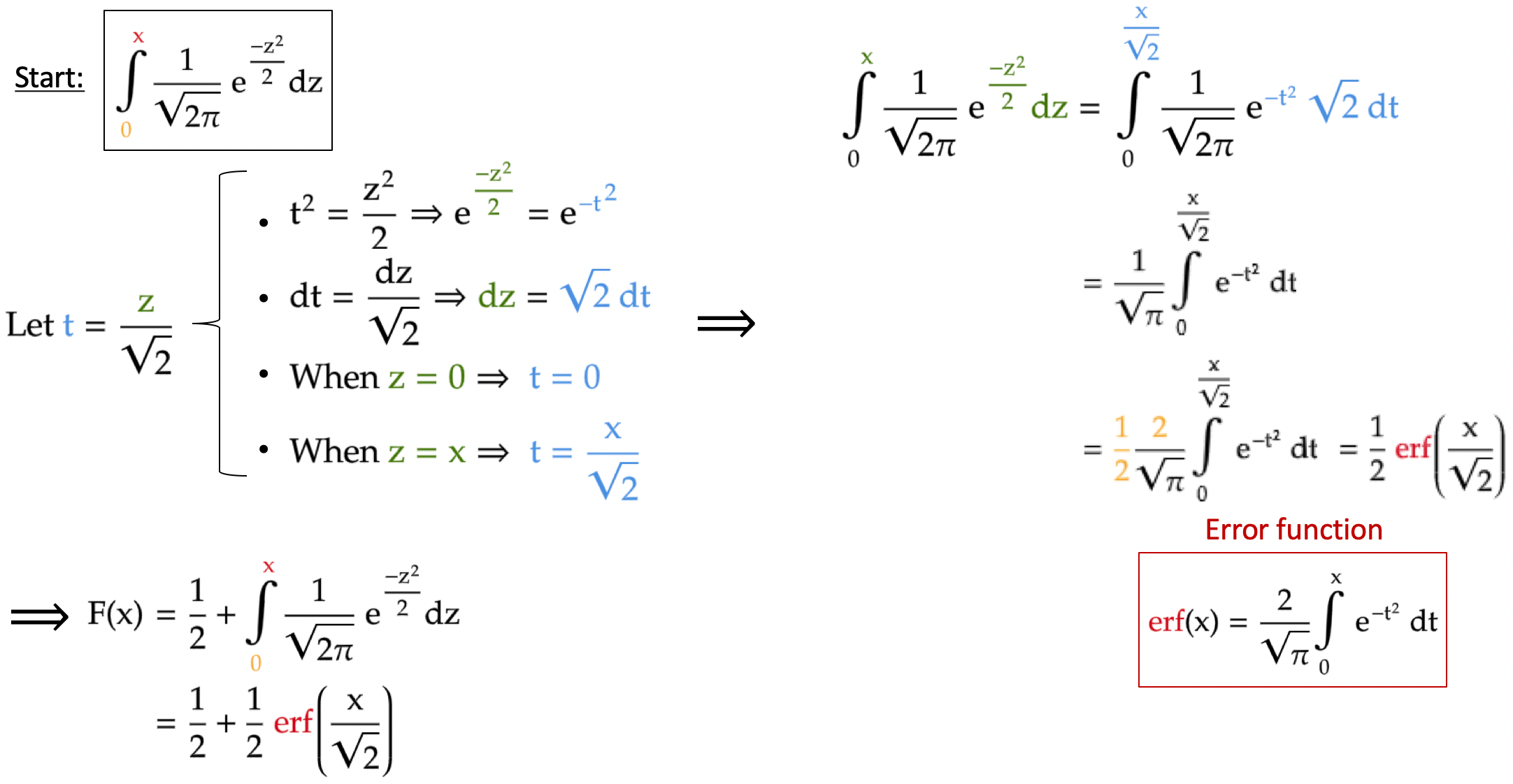Reformulate Gaussian CDF to contain the error function

In my opinion, this modification is totally unnecessary, since the error function, despite its descriptive name, offers little additional insight to the original integral. The sole reason it is still used at all, it seems, stems from historical inertia (and perhaps to intimidate new students of probability). You can learn more about the obscure history of the error function here.

# The inverse CDF of standard Gaussian

Once the CDF of N(0, 1) is found, we can now find its inverse function i.e. the inverse CDF of N(0, 1). The procedure to find the inverse of a function is straightforward enough: write the function as "y in terms of x”, then reformulate the equation so that it becomes “x in terms of y”. In this case, “y” is the cumulative probability / left-side area (which now goes by the variable A) of a Gaussian sample with value “x”. As a result, the inverse CDF of N(0, 1) can be derived below: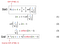Derive inverse standard Gaussian CDF from the CDF

Note that in this derivation, we have to invert the error function (erf) at step 2. This result is erfinv, the inverse of the error function. Hence, once we know how to evaluate this function, we can use it to transform the left-side area (A), which can be generated from a uniform distribution, to get the desired Gaussian sample (x).

# The inverse error function

The bad news: not only can we not evaluate inverse error function directly, but we can’t even write down a formula for the function in the first place!

This is because the original erf function cannot be expressed cleanly as a “y in terms of x” formula, all due to the pesky integration with no closed-form expression at its core (see above formula). As a result, this leaves no way for us to express its inverse function as “x in terms of y”.

The good news: we can still approximate the inverse error function. These approximation methods can get quite complicated — see here for an example — but for now, let’s just use the most common, and likely the easiest method: the Taylor series.

# Taylor series approximation of inverse error function

The Taylor series approximation of a function around a point involves finding the derivatives of the function at that point (see formula below). For the inverse error function, we choose x = 0 as the point to be approximated around, since the erfinv function, and the erf function for that matter, is symmetric around that point.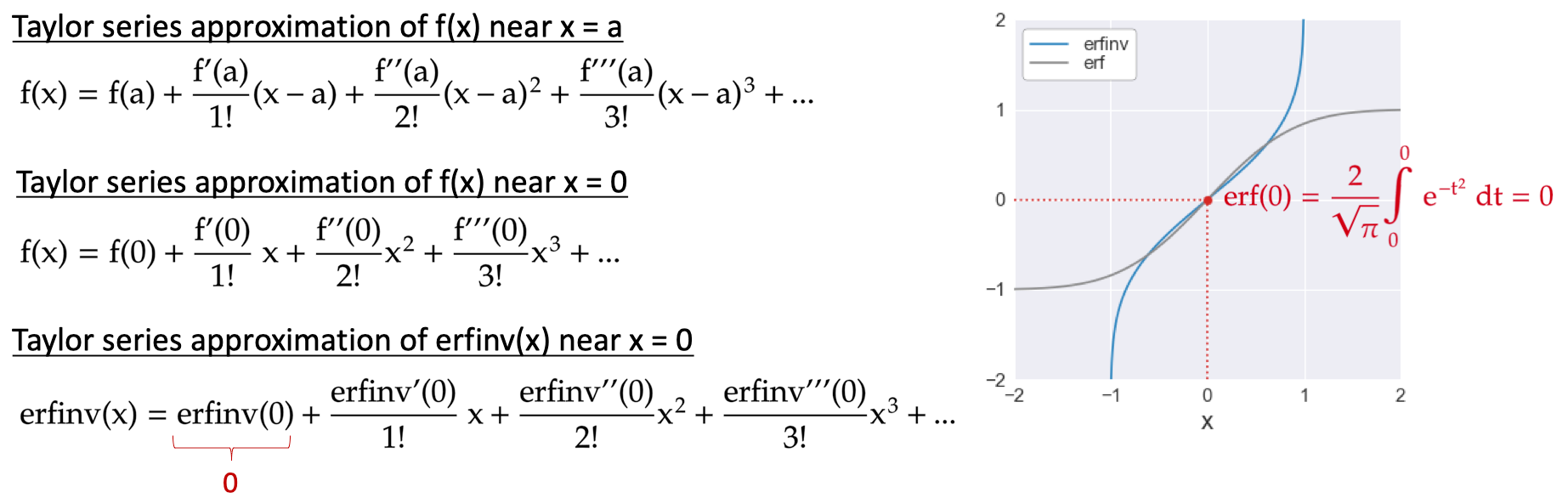Right: erf and erfinv function are both symmetric around the point x = 0

From inspecting the Taylor series approximation of erfinv near x = 0, the first term — erfinv(0) — is zero, since erf(0) = 0 (see right graph above). Therefore, to approximate erfinv, we need to evaluate the subsequent terms of the Taylor series, which are the derivatives of erfinv at zero.

## First derivative of inverse error function

Instead of differentiate the erfinv function directly to get the first derivative, we use a little trick by writing this shockingly obvious equation: erf(erfinv(x)) = x (see left image).

As simple as it might be, differentiating this equation on both side means, per chain rule, we will have an outer derivative of erf(erfinv(x)), and an inner derivative of erfinv(x).

The outer derivative, although appears intimidating at first, turns out to be very easy to differentiate. This is because the variable “x” appears in the upper bound of integration in the error function formula. Therefore, differentiating erf(x) with respect to x will essentially “remove” the integration, as dictated by the first fundamental theorem of calculus.

The inner derivative, which is the first derivative of erfinv that we are after, can then be straightforwardly derived as seen in the left image.

Note that the first derivative of erfinv contains the function itself. However, this is nothing new: many functions share the same behavior (the exponential function for example), and the entire field of differential equation is in fact based on this relationship. Furthermore, the Taylor series approximation only requires us to evaluate the derivatives at x = 0, which turns out to be easy, since erfinv(0) = 0 as shown earlier.

## Higher derivatives of inverse error function

When deriving the higher derivatives of the erfinv, which now goes by the alias E for short, we see that from the second derivative onward, each derivative of order n is a product of:

1. The first derivative (E’) raised to the power of n
2. A polynomial of E, which we call P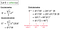First three derivatives of inverse error function (E)

To prove this for certain, we can show that if the derivative of order n of erfinv follows this relationship, then the derivative of order n+1 also follows the same relationship: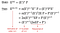Induction step: proof that derivative of order n+1 follows the same structure as that of order n

The above derivation, along with the fact that this relationship holds true for the base case of order 2, means that by mathematical induction, the relationship holds for all derivatives of the erfinv function beyond the second order. Even better, this derivation also allows us to update the polynomial for each derivative (2nEP + P’), simply by using the polynomial P from the derivative right below it.

To demonstrate, let’s code this update rule to find the polynomial for the fourth-order derivative of erfinv, since we already know the polynomial of the third-order derivative: 2 + 8E². Turns out, a polynomial can be represented simply as a Python list, whose element values are the coefficients of the polynomial, as whose indices are the powers of the polynomial.

This polynomial-as-Python-list can then be updated via the following Python function:

In the image above, the result of each step is shown as a Python list on the left, and the polynomial that the list represents is shown on the right. We see that many mathematical operations on the polynomial can be represented as simple list manipulations. For example, the first derivative (step 3) is nothing but:

• Multiplying each list element (`coeff`, which represents the coefficient of each term) by the index of that element (`power`, which represents the power of each term). This means the term 8E², for example, becomes 16E².
• Discarding the constant term in front (by using `[1:]`) so that all powers are now reduced by one. Following the example above, the term 16E² now becomes 16E.

Once the polynomial P is updated for a given derivative, we can re-apply the `update_P` function to it in order to update this polynomial for the next higher derivative.

Furthermore, given that E (erfinv) is zero at x = 0, the only term in each polynomial that will “survive” at x = 0 is the constant term. As a result, each Taylor series coefficient at power n is just the product of that constant term with the first derivative raised to power n, and divided by the factorial of n. After the Taylor series coefficient for that term is found, we update the polynomial P to use for the next term (see bolded section in code block below):

The code block above evaluates the Taylor series coefficient for each term, from the constant term all the way to x⁵⁰. The coefficients for the first few terms of the approximation are shown in the table below:

From the table above, we see that all the even-powered terms have no constant term for their polynomials. Hence, the Taylor series approximation only involves odd-powered terms (x³, x⁵, and so on). Furthermore, a factor of √π/2 is taken out of each term so that the Taylor series approximation formula looks similar to the one found in the Wikipedia page of the error function

## Effect of number of terms in Taylor series approximation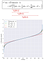Gray lines: odd-degree Taylor approximations (from 1 to 50) of the Gaussian inverse CDF

We can use the above Taylor approximations of erfinv to approximate the inverse CDF of standard Gaussian (see left graph). More specifically, we see that:

• The more terms there are in the Taylor series, the better it approximates the true inverse CDF, which is represented by the blue line on the graph (assuming the scipy.special.erfinv function used to plot it calculates erfinv very accurately). For example, the Taylor series approximation using only the first-power term —T₁, in red— is just a straight line, given the Taylor series itself is just a linear function with respect to A. In contrast, the higher-degree Taylor approximations are more and more curved toward the true inverse CDF.
• However, these higher-degree approximations only approximate well near A = 0.5, where the approximation is anchored on (note that 2A-1 is zero when A = 0.5). In contrast, when A is near 0 or 1, these approximations still significantly under-approximate the value of the true inverse CDF, even when the Taylor series already has many high-power terms (up to x⁵⁰).

Next, we will see how the number of terms in the Taylor series approximation affects the 2-D Gaussian samples that we generate.

# Generate Gaussian samples using inverse transform sampling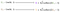Generate x and y coordinates from uniform samples (U₁ and U₂) using inverse transform sampling

In short, to generate our 2-D Gaussian samples, we:

1. Sample independent left-side areas (A) from a uniform distribution (using `numpy.random.uniform` for example).

2. Apply the Taylor series approximation of the inverse Gaussian CDF to each sampled area. This simply gives the corresponding Gaussian sample (x) to that sampled area.

3. For 2-D Gaussian samples, we can first generate standard Gaussian samples for the x-coordinates. Then, we generate standard Gaussian samples for the y-coordinates, preferably using a different uniform generator at the start (one initialized by a different seed, for example).

Below are how these 3 steps are coded in Python to generate 1000 standard Gaussian samples in 2-D:

The `InverseCDF` used in step 2 in the code block above is a class: it encapsulates all the functions mentioned thus far to convert the left-side area A to the Gaussian sample, include the `calculate_sample` method that performs the final conversion:

## Effect of number of terms in Taylor series approximation

Since the x and y coordinates of the 1000 Gaussian samples above are independent from each other, we should expect a nice round blob of Gaussian samples in the 2-D plane (see blue points in graph below).

In contrast, we see that:

• As mentioned earlier, the Taylor series with only first-power term — T₁ — is in fact only a linear function of 2A-1, which itself is a linear function of A. As a result, the Gaussian samples generated from this approximation forms a square (in red), as they are simply linear scalings of the uniformly-sampled left-side areas (black square). In contrast, the more terms the Taylor series approximation of erfinv is, the more and more “blob-like” our generated 2-D Gaussian samples become.
• However, even with many high-power terms, like the T₂₁ Taylor series (samples in green), these approximations still under-sample more extreme values compared to the true inverse Gaussian CDF (in blue). This is consistent with the earlier observation that these Taylor series still under-approximate the inverse Gaussian CDF when the left-side area is near 0 (very small samples) or 1 (very large samples).

# Inverse transform sampling in action: R’s rnorm

## Theory

Given the difficulty of approximating the inverse Gaussian CDF, I was surprised to learn that this is in fact the method that R uses to generate Gaussian samples! Here’s my little investigation to discover it:

• R uses the `rnorm` function to generate Gaussian samples. In the doc for this function, there’s a reference to `RNG`, the random number generator that R uses at the backend of `rnorm`.
• In the doc for `RNG`, the parameter that dictates how Gaussian samples are generated, `normal.kind`, can take different methods (see below). However, the default method is still “Inversion” i.e. the inverse transform sampling. It also helpfully directs the user to the doc for `qnorm` for more information on this inversion sampling method.
• The doc for `qnorm` then refers to the following journal paper for its implementation:
• This paper is thankfully free to read online. However, a quick look within confirms that we are not in Kansas anymore: instead of our simple Taylor series approximation, their approximation of the inverse Gaussian CDF employs what’s called the “minimax rational approximation” of the inverse CDF.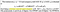Inverse CDF approximation to convert p (cumulative probability) to z (quantile/standard Gaussian sample)

My research for “minimax rational approximation” got really hairy really fast! Therefore, my very best effort of describing this type of approximation is only to explain the two main terms in its name:

• Minimax: we minimize the maximum distance between the approximate function and the real function. In other words, instead of following a set formula like the Taylor series approximation, we have to run an optimization algorithm to make the approximate function as close as possible to the real function.
• Rational: instead of the approximate function taking the form of a polynomial like the Taylor series, it will be a ratio of two polynomials. In the paper, the polynomial A is in the numerator, while the polynomial B is in the denominator of the ratio.

## Implementation

Although these methods sound quite abstract, the actual C implementation of `qnorm` in R’s source code bears them out quite clearly:

From the above code snippet, we see that:

• The approximation of the inverse Gaussian CDF that R uses is indeed a ratio of two polynomials, each of degree 7. The hardcoded coefficients of each polynomial, although quite ugly, are likely the results of the minimax optimization algorithm.
• Furthermore, the polynomials are not written in the typical polynomial form (a+bx+cx²+…), but instead in terms of nested products of (a+bx). This peculiar form is called the Horner’s method, and has been shown to reduce the number of operations to evaluate a polynomial.
• Lastly, in both the paper and the source code, different regions of p (cumulative probability / left-side area) uses a slightly different approximation formula. This is to ensure that the approximation is accurate over the entire range of p (0 to 1). In contrast, our Taylor series approximation is very accurate in the middle (0.5), but much less so at both ends (near 0 and 1).

Nevertheless, this approach till involves approximating the difficult inverse Gaussian CDF. Therefore, in part 2 of the project, we will explore a more direct method to generate 2-D Gaussian samples that do not depend on approximation of the inverse CDF: the Box-Muller transform.

# References

• From my research for the project, the single most helpful guide to derive the Taylor series approximation of the inverse error function is this 1959’s paper by J. R. Philip. The title of the paper: “The function erfinvc θ” refers to the inverse of a related function, the complementary error function (erfc) (see formula below). However, I was able to adapt the derivations therein to the error function and its inverse, as outlined thus far in this blog post.
• The algorithm outlined earlier that underlies R’s `qnorm` goes by the name of AS 241. Although the authors of the algorithm hardly mention how they got the coefficients of their rational minimax approximation, they claim that their algorithm is similar to the algorithm AS 111:
• The paper for AS 111 (Beasley & Spring 1977), then, claims that is is very similar to the algorithm AS 70:
• The paper of AS 70 (Odeh & Evans 1974), in turn, uses the algorithm developed by Evans (1972) for the minimax rational approximation:
• However, this is where I hit a dead-end: Evans (1972) turns out to be someone’s Master thesis at the University of Victoria, one which I could not find anywhere online!
• Therefore, I’m still unclear on how the coefficients that R uses for its rational minimax approximation came about. As a result, if any reader could help me understand exactly where these numbers came from, I would really appreciate it 🙏

## MTI Technology

A place where MTI-ers can publish ideas about new…

## MTI Technology

A place where MTI-ers can publish ideas about new technologies, agile concepts and their working experiences

Written by

## Khanh Nguyen

Data scientist based in Ho Chi Minh City, Vietnam | dknguyen.com## MTI Technology

A place where MTI-ers can publish ideas about new technologies, agile concepts and their working experiences

Medium is an open platform where 170 million readers come to find insightful and dynamic thinking. Here, expert and undiscovered voices alike dive into the heart of any topic and bring new ideas to the surface.

Follow the writers, publications, and topics that matter to you, and you’ll see them on your homepage and in your inbox.

If you have a story to tell, knowledge to share, or a perspective to offer — welcome home. It’s easy and free to post your thinking on any topic.